# 基于Snorkel弱监督和ULMFiT迁移学习的微博情感分类ezpod 2019-08-22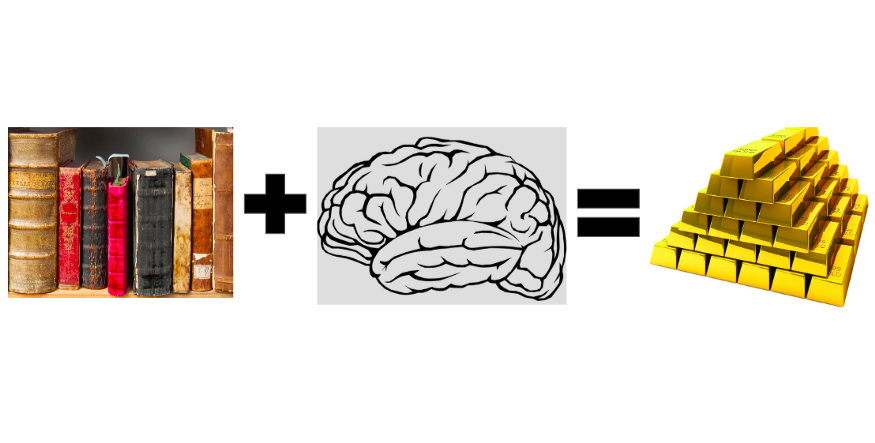• 采集一小批已标注样本（~600）
• 使用弱监督利用大量未标注样本生成训练集
• 使用一个大型预训练语言模型进行迁移学习

## 弱监督• 硬编码规则：通常使用正则表达式
• 语义规则：例如，使用spaCy的依存树
• 远程监督：使用外部知识库
• 有噪声人工标注：众包标注
• 外部模型：包含有价值信号的第三方模型

``````# Set voting values.
ABSTAIN = 0
POSITIVE = 1
NEGATIVE = 2# Detects common conspiracy theories about jews owning the world.

def jews_symbols_of_globalism(tweet_text):
return POSITIVE if re.search(GLOBALISM, tweet_text) else ABSTAIN``````

• 灵活：需要更新模型时，只需要更新标注函数、重新生成训练集和分类器即可
• 提高召回率：判别模型将提供优于弱监督模型的泛化能力，因此可以提高召回率

## 迁移学习和ULMFiT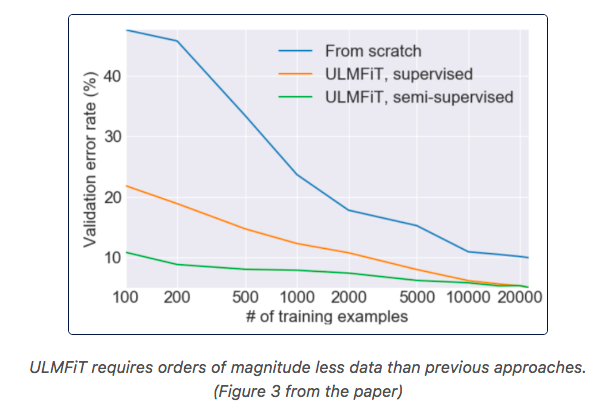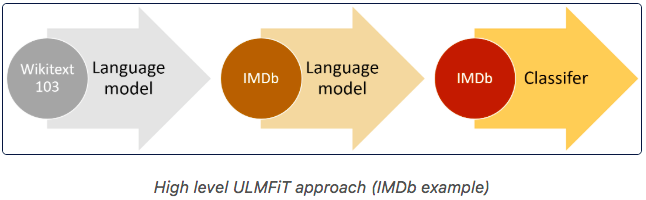UMLFiT的最大优势在于：

• 只需要100个标注，就可以达到使用100倍的数据训练的效果
• Fastai的API非常易用，这个教程非常好
• 得到的Pytorch模型可以在生产环境中部署

## 第一步：数据采集和目标设定``````DATA_PATH = "../data"
train.shape, LF_set.shape, test.shape

>> ((24738, 6), (733, 7), (438, 7))``````

## 第二部：使用Snorkel构建训练数据集

``````# Common insults against jews.
INSULTS = r"\bjew (bitch|shit|crow|fuck|rat|cockroach|ass|bast(a|e)rd)"

def insults(tweet_text):
return POSITIVE if re.search(INSULTS, tweet_text) else ABSTAIN``````

``````# If tweet author is jewish then it's likely not anti-semitic.
JEWISH_AUTHOR = r"((\bI am jew)|(\bas a jew)|(\bborn a jew)"

def jewish_author(tweet_tweet):
return NEGATIVE if re.search(JEWISH_AUTHOR, tweet_tweet) else ABSTAIN``````

``````# We build a matrix of LF votes for each tweet
LF_matrix = make_Ls_matrix(LF_set, LFs)

# Get true labels for LF set
Y_LF_set = np.array(LF_set['label'])

display(lf_summary(sparse.csr_matrix(LF_matrix),
Y=Y_LF_set,
lf_names=LF_names.values()))``````• Emp. Accuracy：正确的标注函数预测比例。所有的标注函数都应当不低于0.5。
• Coverage：覆盖率，被成功标注（正或负）的样本占比。需要尽可能提高这个值，同时保持良好的准确率。
• Polarity：标注函数返回值的极性
• Overlaps & Conflicts：一个标注函数与其他标注函数的重叠与冲突情况。标注模型将使用这些信息来估算每个标注函数的准确率。

``````label_coverage(LF_matrix)
>> 0.8062755798090041``````

``````from metal.label_model.baselines import MajorityLabelVotermv = MajorityLabelVoter()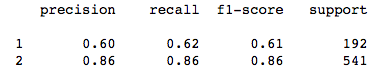``````Ls_train = make_Ls_matrix(train, LFs)

# You can tune the learning rate and class balance.
label_model = LabelModel(k=2, seed=123)
label_model.train_model(Ls_train, n_epochs=2000, print_every=1000,
lr=0.0001,
class_balance=np.array([0.2, 0.8]))````````````# To use all information possible when we fit our classifier, we can
# actually combine our hand-labeled LF set with our training set.

Y_train = label_model.predict(Ls_train) + Y_LF_set``````

1. 遍历标注集样本，获取新标注函数的思路
2. 将新的标注函数加入标注矩阵，准确率不低于50%，并尽可能提高准确率及覆盖率
3. 定期使用投票模型更新标注函数集
4. 如果投票模型不够好，可以改善标注函数并返回第1步重复
5. 一旦投票模型有效，那么就在训练集上跑标注函数，应当至少达到60%的覆盖率
6. 接着训练标注模型
7. 我在验证标注模型时，使用了我的训练集并打印出100个最反犹太tweet的100个最不反犹太的tweet来确保其工作正常

Snorkel的提示：

• 关于LF准确率：在弱监督步骤，我们目标是高精度，所有的标注函数在标注集上应当至少达到50%的准确率。如果能达到75%甚至更高的话，那就再好不过了
• 关于LF覆盖率：在训练集上应当至少达到65%的覆盖率
• 如果你不是领域专家，那么当你标记初始的600个数据后将得到新的标注函数思路

## 第三步：训练分类模型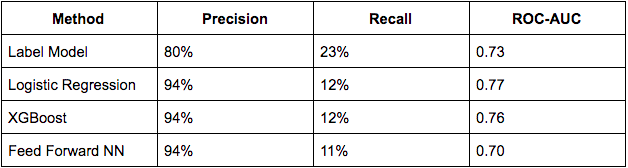``````data_lm = TextLMDataBunch.from_df(train_df=LM_TWEETS, valid_df=df_test, path="")
learn_lm = language_model_learner(data_lm, pretrained_model=URLs.WT103_1, drop_mult=0.5)``````

``learn_lm.unfreeze()``

``````for i in range(20):
learn_lm.fit_one_cycle(cyc_len=1, max_lr=1e-3, moms=(0.8, 0.7))

``````learn_lm.predict("i hate jews", n_words=10)
>> 'i hate jews are additional for what hello you brother . xxmaj the'
learn_lm.predict("jews", n_words=10)
>> 'jews out there though probably okay jew back xxbos xxmaj my'``````

``````# Classifier model data
data_clas = TextClasDataBunch.from_df(path = "",
train_df = df_trn,
valid_df = df_val,
vocab=data_lm.train_ds.vocab,
bs=32,
label_cols=0)learn = text_classifier_learner(data_clas, drop_mult=0.5)
learn.freeze()``````

``````learn.lr_find(start_lr=1e-8, end_lr=1e2)
learn.recorder.plot()``````

``````learn.fit_one_cycle(cyc_len=1, max_lr=1e-3, moms=(0.8, 0.7))
learn.freeze_to(-2)
learn.fit_one_cycle(1, slice(1e-4,1e-2), moms=(0.8,0.7))
learn.freeze_to(-3)
learn.fit_one_cycle(1, slice(1e-5,5e-3), moms=(0.8,0.7))
learn.unfreeze()
learn.fit_one_cycle(4, slice(1e-5,1e-3), moms=(0.8,0.7))``````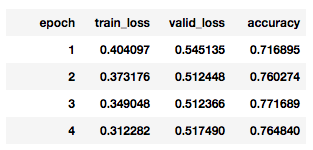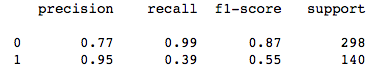## 模型拾趣

``````learn.predict("george soros controls the government")
>> (Category 1, tensor(1), tensor([0.4436, 0.5564]))learn.predict("george soros doesn't control the government")
>> (Category 0, tensor(0), tensor([0.7151, 0.2849]))``````

``````learn.predict("fuck jews")
>> (Category 1, tensor(1), tensor([0.1996, 0.8004]))learn.predict("dirty jews")
>> (Category 1, tensor(1), tensor([0.4686, 0.5314]))``````

``````learn.predict("Wow. The shocking part is you're proud of offending every serious jew, mocking a religion and openly being an anti-semite.")
>> (Category 0, tensor(0), tensor([0.9908, 0.0092]))``````

``````learn.predict("my cousin is a russian jew from ukraine-  i'm so glad they here")
>> (Category 0, tensor(0), tensor([0.8076, 0.1924]))learn.predict("at least the stolen election got the temple jew shooter off the drudgereport. I ran out of tears.")
>> (Category 0, tensor(0), tensor([0.9022, 0.0978]))``````

## 弱监督是否真有作用？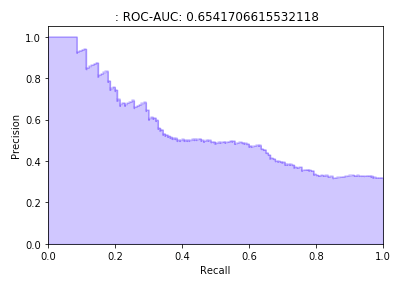6326人浏览
2020-07-13

2017深度学习NLP进展与趋势
5376人浏览
2017-12-17 15:23:02
NLP中的迁移学习
2235人浏览
2018-08-21 18:27:44

1479人浏览
2017-09-15 18:08:20

3418人浏览
2018-12-12 13:20:51

2092人浏览
2018-12-16 19:13:24
2018年深度学习的主要进步
2183人浏览
2018-12-30 09:20:25

365人浏览
2020-07-27 12:30:13
0
0
1202